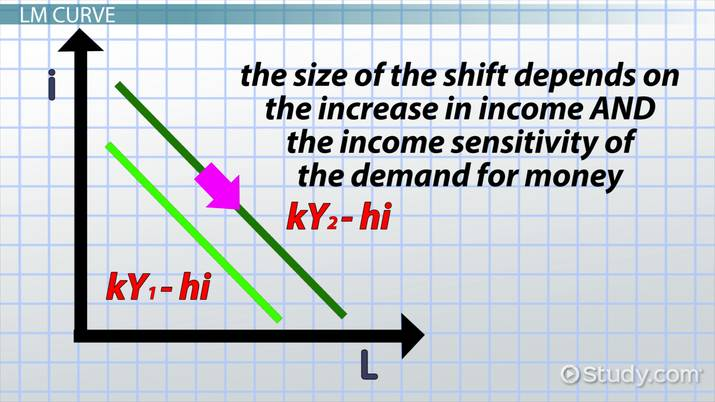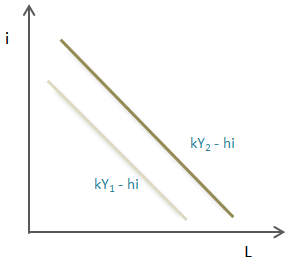Business Courses / Course / Chapter

# LM Curve in Macroeconomics: Definition & Equation

Lesson Transcript
Instructor: Martin Gibbs

Martin has 21 years experience in Information Systems and Information Technology, has a PhD in Information Technology Management, and a master's degree in Information Systems Management. He is an adjunct professor of computer science and computer programming.

In macroeconomics, the LM curve refers to a visual representation of the liquidity of money. Learn more about the definition of the LM curve, definitions LM and equilibrium, the LM equation, and the LM curve on a graph. Updated: 10/14/2021

## What Is LM?

In macroeconomics terms, LM refers to the liquidity of money. As interest rates increase, the demand for money decreases. LM is really part of a larger model, the IS-LM model, where IS-LM stands for Investment Saving - Liquidity Preference Money Supply. These large words are basically just used to model money and income in an economy.

The models are used to define points of equilibrium, or balance; in other words, intersecting values where the demanded money equals the amount available to invest. While the formulas are a little more complex, it boils down to the basic economic analysis of supply versus demand.An error occurred trying to load this video.

Try refreshing the page, or contact customer support.

Coming up next: Macroeconomic Equilibrium: Definition & Overview

### You're on a roll. Keep up the good work!

Replay
Your next lesson will play in 10 seconds
• 0:00 What Is LM?
• 0:46 LM Equation
• 1:56 LM Curve
• 3:31 LM Curve on a Graph
• 4:08 Lesson Summary
Save Save

Want to watch this again later?

Timeline
Autoplay
Autoplay
Speed Speed

## LM Equation

The LM equation calculates the demand for money, and the equation is represented here:

L = k * Y - h * I

• L = Demand for Real Money
• k = Income Sensitivity of Demand for Real Money
• Y = Income
• h = Interest Sensitivity of Demand for Real Money
• i = Interest Rate

When we talk about real money, we aren't comparing real cash to Monopoly money. Instead, real money is adjusted for inflation. This tells us the true purchasing ability of money, or in essence, what your money can get you. The demand for money also depends on income. The more you make, the more you spend, or save in offshore accounts. It also depends on the interest rate, since people also leverage their money through investing.

We're subtracting the interest rate. As interest rates rise, people tend to invest their money.

The variables k (income sensitivity) and h (interest sensitivity) are modifiers that are added to income and the interest rates and will have value greater than zero. When talking about sensitivity in this case, we're talking about the likelihood of change.

## LM Curve

Before we can map out the full LM curve, let's take a look at the demand for money, the L in the equation, in graph form. The LM equation can be used to create a straight line, much as the standard math formula (y = mx + b).

We'll put the interest rate on the y-axis, since this is the independent variable; we'll put L on the x-axis, since this is the demand for money. When interest rates go down, so does the demand for money. Again, we're talking about real money, not Monopoly money or pre-inflation dollars.

This graph is showing two sample calculations for L as plotted on the demand for money graph.As national income increases, the demand curve shifts upward and outward, toward L on the graph. The size of the shift depends on the increase in income and the income sensitivity of the demand for money.

To unlock this lesson you must be a Study.com Member.

### Register to view this lesson

Are you a student or a teacher?

Back

### Resources created by teachers for teachers

Over 30,000 video lessons & teaching resources‐all in one place.Video lessonsQuizzes & WorksheetsClassroom IntegrationLesson Plans

I would definitely recommend Study.com to my colleagues. It’s like a teacher waved a magic wand and did the work for me. I feel like it’s a lifeline.

Jennifer B.
TeacherCreate an account to start this course today
Used by over 30 million students worldwide